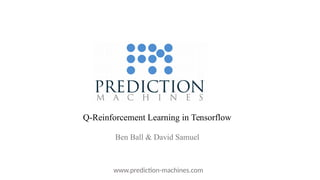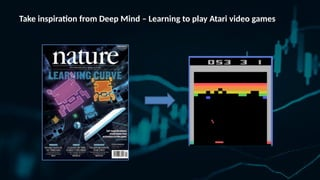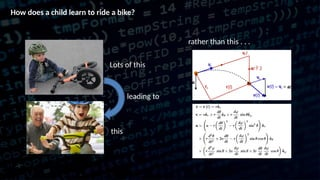Diese Präsentation wurde erfolgreich gemeldet.

# TensorFlow and Deep Learning Tips and Tricks

Anzeige
Anzeige
Anzeige
Anzeige
Anzeige
Anzeige
Anzeige
Anzeige
Anzeige
Anzeige
Anzeige×

1 von 33 Anzeige

# TensorFlow and Deep Learning Tips and Tricks

Presented at https://www.meetup.com/TensorFlow-and-Deep-Learning-Singapore/events/241183195/ . Tips and Tricks for using Tensorflow with Deep Reinforcement Learning.

Presented at https://www.meetup.com/TensorFlow-and-Deep-Learning-Singapore/events/241183195/ . Tips and Tricks for using Tensorflow with Deep Reinforcement Learning.

Anzeige
Anzeige

### TensorFlow and Deep Learning Tips and Tricks

1. 1. Q-Reinforcement Learning in Tensorflow Ben Ball & David Samuel www.prediction-machines.com
2. 2. Take inspiration from Deep Mind – Learning to play Atari video games
3. 3. How does a child learn to ride a bike? Lots of this leading to this rather than this . . .
4. 4. Machine Learning vs Reinforcement Learning  No supervisor  Trial and error paradigm  Feedback delayed  Time sequenced  Agent influences the environment Agent Environment Action atState St Reward rt & Next State St+1 Good textbook on this by Sutton and Barto - st, at, rt, st+1,at+1,rt+1,st+2,at+2,rt+2, …
5. 5.   Mathematical formulation of RL
6. 6. S = A = T: S4(t) → S1(t+1) = 0.5 a1 1 r(t)=1 Markov Decision Process (MDP) Example: reward
7. 7. Markov Decision Process (MDP) Example: Grid World 20 states 4 actions: Game involves moving from a starting state (box) to one occupied by a yellow star in as few steps as possible
8. 8. -1 -1 -1 -1 -1 -1 -2 -2 -2 -2 -2 -2 -2 -2 -3 -3 -3 -4 Markov Decision Process (MDP) Example: Grid World Optimal Policy Value function
9. 9. An aside – neural networks What is important to remember when creating one? It is really just a way to represent a nonlinear many-to-many function mapping. It takes m inputs, giving n outputs. The outputs are a nonlinear transformation of the inputs. For this network to learn this nonlinear function, it must be able to represent the superset of all requisite functions to calculate that mapping. t-1 t
10. 10. Markov Decision Process (MDP) Markov property The conditional probability distrubution of future states depends only on the present state, not on the sequence of events that preceded it. t-2 t-1 t Q: At a single time step (as a state), are you able to see the velocity and acceleration?
11. 11. Markov Decision Process (MDP) Markov property The conditional probability distrubution of future states depends only on the present state, not on the sequence of events that preceded it. t-2 t-1 t Super state A : No, you are not. For us to learn a function that utilizes velocity and accelaration, AND for this to be Markov, we must create a synthetic super state as the single state of the system. Composed of the last two time steps
12. 12. Putting it all together • Q(s,a) is the Q function that gives the value of taking a specific action from a specific state • These states,actions,next-states must be markov • Deepnets are good at learning complex nonlinear functions, such as the Q function • (Optimizing the Q function is done through the bellman equation, which is used to calculate the reward) • Once we learn the Q function with our deepnet, we apply it as the optimal policy t-1 t
13. 13. Application to Transactional Markets
14. 14. State transitions of lattice simulation of mean reversion: Short LongFlat Spreadpricemappedontolatticeindex i = 0 i = -1 i = -2 i = 1 i = 2 sell buy These map into: (State, Action, Reward) triplets used in the QRL algorithm
15. 15. http://www.prediction-machines.com/blog/ - for demonstration As per the Atari games example, our QRL/DQN plays the trading game … over-and-over
17. 17. + Input FC ReLU FC ReLU Functional pass-though Output + Input FC ReLU FC ReLU Functional pass-though Output Double Dueling DQN (vanilla DQN does not converge well but this method works much better) target networktraining network lattice position (long,short,flat) state value of Buy value of Sell value of Do Nothing
18. 18. DDDQN and Tensorflow
19. 19. Overview 1. DQN - DeepMind, Feb 2015 “DeepMindNature” http://www.davidqiu.com:8888/research/nature14236.pdf a. Experience Replay b. Separate Target Network 2. DDQN - Double Q-learning. DeepMind, Dec 2015 https://arxiv.org/pdf/1509.06461.pdf 3. Prioritized Experience Replay - DeepMind, Feb 2016 https://arxiv.org/pdf/1511.05952.pdf 4. DDDQN - Dueling Double Q-learning. DeepMind, Apr 2016 https://arxiv.org/pdf/1511.06581.pdf
20. 20. Enhancements Experience Replay Removes correlation in sequences Smooths over changes in data distribution Prioritized Experience Replay Speeds up learning by choosing experiences with weighted distribution Separate target network from Q network Removes correlation with target - improves stability Double Q learning Removes a lot of the non uniform overestimations by separating selection of action and evaluation Dueling Q learning Improves learning with many similar action values. Separates Q value into two : state value and state- dependent action advantage
21. 21. Install Tensorflow My installation was on CentOS in docker with GPU*, but also did locally on Ubuntu 16 for this demo. *Built from source for maximum speed. CentOS instructions were adapted from: https://blog.abysm.org/2016/06/building-tensorflow-centos-6/ Ubuntu install was from: https://www.tensorflow.org/install/install_sources
22. 22. Tensorflow - what is it A computational graph solver
23. 23. Tensorflow key API Namespaces for organizing the graph and showing in tensorboard with tf.variable_scope('prediction'): Sessions with tf.Session() as sess: Create variables and placeholders var = tf.placeholder('int32', [None, 2, 3], name='varname’) self.global_step = tf.Variable(0, trainable=False) Session.run or variable.eval to run parts of the graph and retrieve values pred_action = self.q_action.eval({self.s_t['p']: s_t_plus_1}) q_t, loss= self.sess.run([q['p'], loss], {target_q_t: target_q_t, action: action})
24. 24. Tensorflow tips and tricks Injecting data into tensorboard agent.inject_summary({'average.reward': avg_reward, 'average.loss': avg_loss, 'average.q': avg_q}, step) def inject_summary(self, tag_dict, step): summary_str_lists = self.sess.run([self.summary_ops[tag] for tag in tag_dict.keys()], { self.summary_placeholders[tag]: value for tag, value in tag_dict.items() }) for summary_str in summary_str_lists: self.writer.add_summary(summary_str, step) with tf.variable_scope('summary'): scalar_summary_tags = ['average.reward', 'average.loss', 'average.q', ] self.summary_placeholders = {} self.summary_ops = {} for tag in scalar_summary_tags: self.summary_placeholders[tag] = tf.placeholder('float32', None, name=tag.replace(' ', '_')) self.summary_ops[tag] = tf.summary.scalar("%s-%s" % (self.env_name, tag), self._placeholders[tag])
25. 25. Tensorflow tips and tricks Clean design
26. 26. Tensorflow tips and tricks Follow Common Patterns http://www.tensorflowpatterns.org/patterns/ • Cloud ML export • Evaluate function • Feed dict as positional arg • Init functions • Loss operation • PEP-8 style for python • Prepare, train, evaluate • Save model function • Summaries operation • Train function • Use default graph
27. 27. Tensorflow tips and tricks Etc • Collect all params in one place • Allows you to easily reconfigure • Can easily do grid search optimization • Avoid all magic numbers • Can compare with other papers and results easily • Keep as much as you can in c++ code • Use numpy and pandas dataframes for matrix comptutation • Via py_func • Use tensorflow functions when possible
28. 28. Trading-Gym + Trading-Brain Architecture Runner warmup() train() run() Children class Agent act() observe() end() DQN Double DQN A3C Abstract class Memory add() sample() Brain train() predict() Data Generator Random Walks Deterministic Signals CSV Replay Market Data Streamer Single Asset Multi Asset Market Making Environment render() step() reset() next() rewind() Trading-Gym - OpenSourced Trading-Brain – On Github
29. 29. Trading-Gym https://github.com/Prediction-Machines/Trading-Gym Open sourced Modelled after OpenAI Gym. Compatible with it. Contains example of DQN with Keras Contains pair trading example simulator and visualizer
30. 30. Prediction Machines release of Trading-Gym environment into OpenSource - - demo - -
31. 31. Trading-Brain https://github.com/Prediction-Machines/Trading-Brain Two rich examples Contains the Trading-Gym Keras example with suggested structuring examples/keras_example.py Contains example of Dueling Double DQN for single stock trading game examples/tf_example.py
32. 32. References Much of the Brain and config code in this example is adapted from devsisters github: https://github.com/devsisters/DQN-tensorflow Our github: https://github.com/Prediction-Machines Tensorflow patterns: http://www.tensorflowpatterns.org Our blog: http://prediction-machines.com/blog/ Our job openings: http://prediction-machines.com/jobopenings/